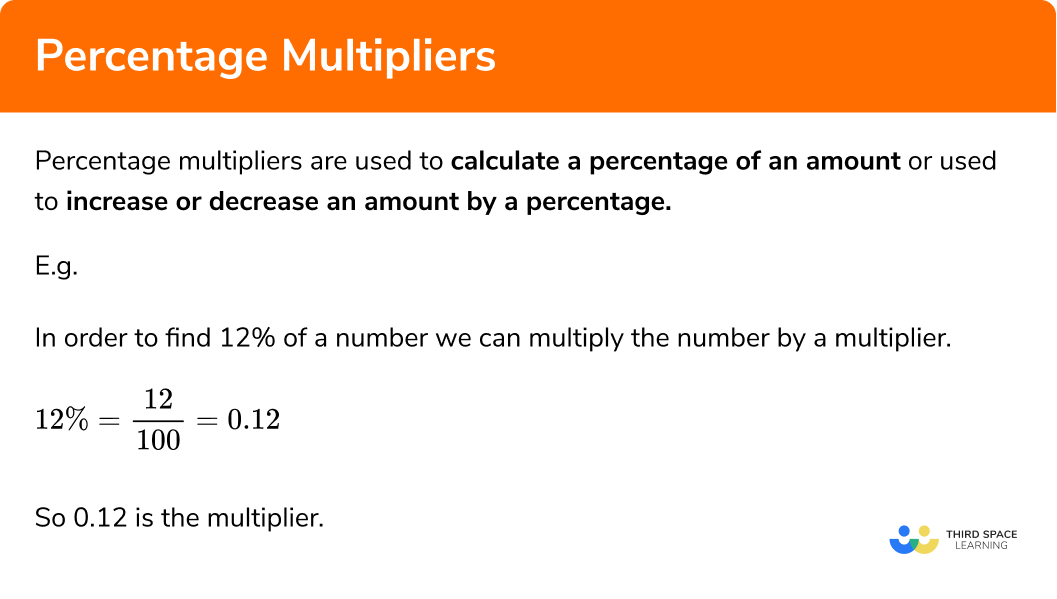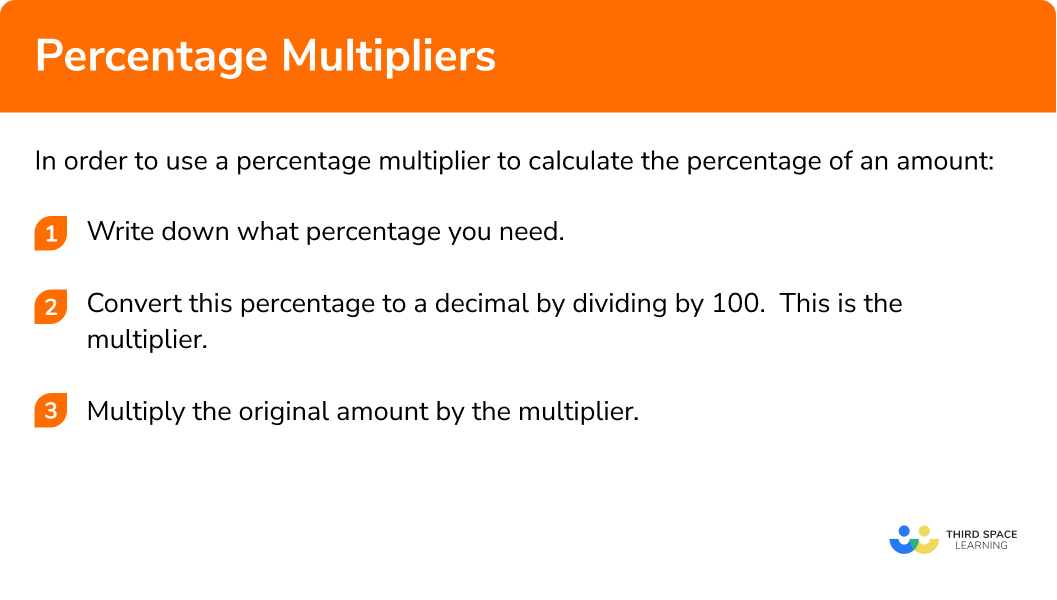GCSE Maths Number Percentages

Percentage Multipliers

# Percentage Multipliers

Here we will learn about about using a percentage multiplier including how to find the single multiplier from a percentage and use the single multiplier to answer percentage questions.
There are also percentage multiplier worksheets based on Edexcel, AQA and OCR exam questions, along with further guidance on where to go next if you’re still stuck.

## What is a percentage multiplier?

A percentage multiplier is a number which is used to calculate a percentage of an amount or used to increase or decrease an amount by a percentage.

E.g.

In order to find 12% of a number we can multiply the number by a multiplier:

$12\% = \frac{12}{100} = 0.12$

So 0.12 is the multiplier.

### What is a percentage multiplier?These questions will often involve interest rates in financial situations such as simple interest or compound interest.  It is sometimes referred to as the multiplier method.

## How to find a decimal multiplier from a percentage

In order to write a decimal multiplier from a percentage:

1. Write down the percentage
2. Convert this percentage to a decimal by dividing by 100 – this is the multiplier
3. Multiply the original amount by the multiplier

### Explain how to write a decimal multiplier from a percentage in 3 steps## Percentage multiplier examples

### Example 1: finding the decimal multiplier

What is the decimal multiplier for 58 \%?

1. Write down the percentage required

$58 \%$

2Convert the percentage to a decimal by dividing by 100

$58\% = \frac{58}{100} = 0.58$

The decimal multiplier is 0.58.

### Example 2: finding the decimal multiplier

What is the decimal multiplier for 26%?

$26 \%$

$26\% = \frac{26}{100} = 0.26$

The decimal multiplier is 0.26.

### Example 3: finding the decimal multiplier

What is the decimal multiplier for 4.5%?

$4.5 \%$

$4.5\% = \frac{4.5}{100} = 0.045$

The decimal multiplier is 0.045.

## How to use a percentage multiplier to calculate the percentage of an amount

In order to use a percentage multiplier to calculate the percentage of an amount:

1. Write down what percentage you need
2. Convert this percentage to a decimal by dividing by 100; this is the decimal multiplier
3.  Multiply the original amount in the question by the decimal multiplier

### Example 4: finding the percentage of an amount

Work out 34% of £700

$34 \%$

$34\% = \frac{34}{100} = 0.34$

What is the original amount? 700

What is the decimal multiplier? 0.34

$700\times0.34=238$

### Example 5: finding the percentage of an amount

Work out 8% of £650

$8 \%$

$8\% = \frac{8}{100} = 0.08$

What is the original amount? 650

What is the decimal multiplier? 0.08

$650\times0.08=52$

### Example 6: finding the percentage of an amount

Work out 15.6% of 200 kg

$15.6 \%$

$15.6\% = \frac{15.6}{100} = 0.156$

What is the original amount? 200

What is the decimal multiplier? 0.156

$200\times0.156=31.2$

### Example 7: calculating a percentage increase

Increase £320 by 25%

$100\%+25\%=125\%$

$125\% = \frac{125}{100} = 1.25$

What is the original amount? 320

What is the decimal multiplier? 1.25

$320\times1.25=400$

### Example 8: calculating a percentage decrease

Decrease £600 by 7%

$100\%-7\%=93\%$

$93\% = \frac{93}{100} = 0.93$

What is the original amount? 600

What is the decimal multiplier? 0.93

$600\times0.93=558$

### Common misconceptions

• Decimal multipliers greater than 1

If you need the decimal multiplier of 135% it would be 1.35, so multipliers can in fact be greater than 1.

$135\% = \frac{135}{100} = 1.35$

• Take care to remember pence when you are working with money

An answer of £43.7 should be written as £43.70.  You need two decimal places for the pence part of the answer.

Percentage multipliers is part of our series of lessons to support revision on percentages. You may find it helpful to start with the main percentages lesson for a summary of what to expect, or use the step by step guides below for further detail on individual topics. Other lessons in this series include:

### Practice percentage multiplier questions

1. What is the decimal multiplier of 61\% ?

0.616.106100.06161\% = \frac{61}{100} = 0.61

So 0.61 is the multiplier.

2. What is the decimal multiplier of 5\% ?

0.55.005000.055\% = \frac{5}{100} = 0.05

So 0.05 is the multiplier.

3. What is the decimal multiplier of 18.3\% ?

0.1831.8318.30.018318.3\% = \frac{18.3}{100} = 0.183

So 0.183 is the multiplier.

4. Work out 29\% of £400

\pounds 371\pounds 116\pounds 29\pounds 42929\% = \frac{29}{100} = 0.29

So 0.29 is the multiplier.

0.29 \times 400 = 116

5. Work out 9\% of 450 km?

45.0 km40.5 km441 km50.0 km9\% = \frac{9}{100} = 0.09

So 0.09 is the multiplier.

0.09 \times 450 = 40.5

6. Work out 14.8\% of 560 kg?

8.288 kg412 kg148 kg82.88 kg14.8\% = \frac{14.8}{100} = 0.148

So 0.148 is the multiplier.

0.148 \times 560 = 82.88

### Percentage multiplier GCSE questions

1.  Write 61\% as a decimal

(1 mark)

61\% = \frac{61}{100} = 0.61

0.61

(1)

2.  Work out 38\% of 600 kg

(2 marks)

0.38 × 600

(1)

= 228 kg

(1)

3.  Fiona is booking a holiday.

The holiday costs £700 .

She pays a 15\% deposit.

Work out how much she has left to pay.

(2 marks)

100\% \hspace{1mm}- 15\% = 85\%=0.85

0.85 × 700

(1)

\pounds 595

(1)

## Learning checklist

You have now learned how to:

• Find the decimal multiplier of a percentage
• Interpret percentages and percentage changes as a decimal
• Use the decimal multiplier to work out the percentage of an amount
• Interpret percentages multiplicatively

## Still stuck?

Prepare your KS4 students for maths GCSEs success with Third Space Learning. Weekly online one to one GCSE maths revision lessons delivered by expert maths tutors.

Find out more about our GCSE maths tuition programme.